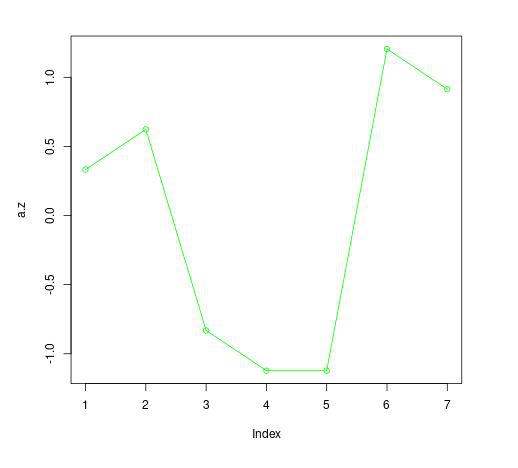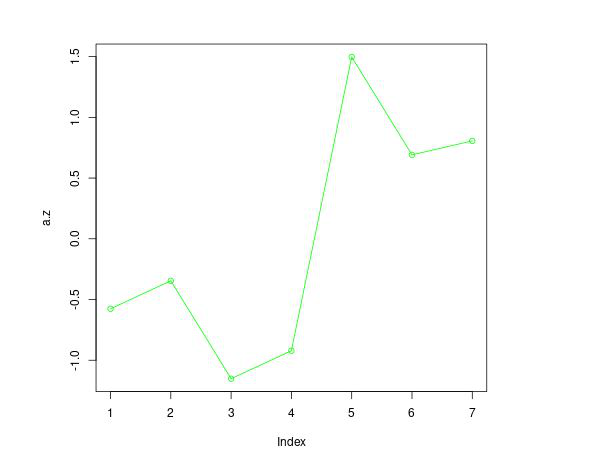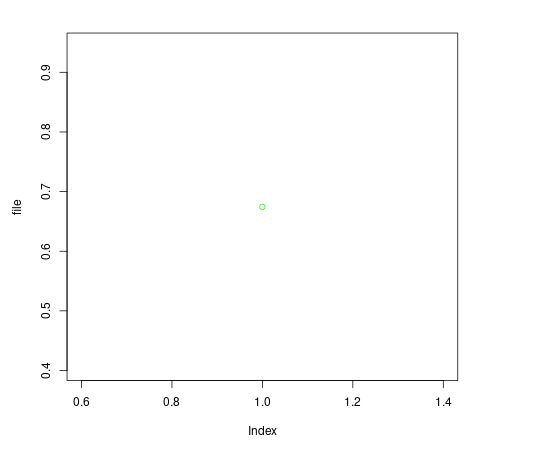Related Articles

# Plot Z-Score in R

• Last Updated : 24 Jun, 2021

R supports powerful tools to plot z-score according to a given p-value. Thus, to learn about the z score we should know about the p-value. p-value and z scores are called statistical parameters and are used to make statistical calculations.

p-value is the probability of obtaining results at least as extreme as the observed result. Just like probability p-values lie between 0 and 1. If the Null hypothesis of a study comes out to be true then the p-value or calculated probability is the probability of finding the more extreme results.

z-score describes a value’s relationship to the mean of the group values. Let us take an example to understand the concept of z-score properly:

Consider a case of a class of 25 students. After the exams, the mean score of the class comes out to be 45. If we want to know whether a person who has scored 75 marks in the exam is among 10% of the scorers. In starting, it may seem to be a very tedious calculation. But by knowing the concept of z-scores it can become fairly easy.

The formula for calculating z-score:• Standard deviation means how far the result is from the average value.
• Now a z score of 1 denotes that the observation is at a distance of one standard deviation towards right from the center.
• Similarly, a z score of -1 tells us that the observation is one standard deviation left from the center.

### Method 1: Naive approach

Approach:

• Create a vector and assign various values to it.
• Find the mean of the vector using function mean().
• Find the standard deviation using function sd().
• Subtract the mean value from the observation and divide the resultant with standard deviation.
• The vector obtained will have the required Z-score values.
• Now simply plot it.

Example 1:

## R

 `# create vector``a <- ``c``(9, 10, 12, 14, 5, 8, 9)` `# find mean``mean``(a)` `# find standard deviation``sd``(a)` `# calculate z``a.z <- (a - ``mean``(a)) / ``sd``(a)` `# plot z-score``plot``(a.z, type=``"o"``, col=``"green"``)`

Output:Example 2:

## R

 `# create vector``a <- ``c``(7, 9, 2, 4, 25, 18, 19)` `# find mean``mean``(a)` `# find standard deviation``sd``(a)` `# calculate z-score``a.z <- (a - ``mean``(a)) / ``sd``(a)` `# plot z-score``plot``(a.z, type=``"o"``, col=``"green"``)`

Output:### Method 2: Using qnorm()

If we are given a p-value and our value is 0.70 then this means that it will be a point below which there are 80% of observations and 20% of observations lie above it. The easiest way for finding a z score if a p-value is given is to use qnorm() function. It takes the p-value as an argument and gives the z score as output.

Syntax:

`qnorm(p-value)`

Approach:

• Call qnorm() function with required p-value
• Plot z-score with the value so obtained

Example :

## R

 `set <- ``qnorm``(0.75)` `plot``(set, type=``"o"``, col=``"green"``)`

Output:My Personal Notes arrow_drop_up